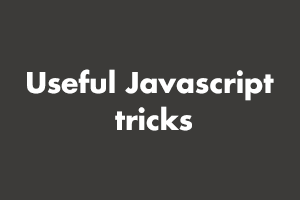Author - StudySection Post Views - 95 views# Useful Javascript tricks

## Javascript tricks

1. Get Unique Values: We can create an array of unique javascript values by using the Set and spread operator as shown below:
```var array = [1,1,3,4,5,3,5,6,8,9,9,9] var unique_array = [...new Set(array)]; console.log(array); // [1,3,4,5,6,8,9]```
2. Convert String to Number: You can convert a string to number using unary operator “+” as shown below.
```var string = “23”; console.log(+string); // 23 var new_string = “Hello”; console.log(+new_string); // Nan```
Note that this operator will only work for number strings.
3. Convert a number to string: To convert a number to string just add empty string with number as shown below:
```var number = 23; number = number + “”; console.log(typeof number)```
4. Short circuit conditions: Conditional code can be reduced using the below trick.
```if(condition){ function(); }```
Instead of the above, you can use the below code which will reduce lines of code and time.
`condition && function()`
5. Concat two arrays using spread operator:
```var array1 = [1, 2, 3, 4, 5, 6, 7]; var array2 = [8, 9, 10, 11, 12]; var array3 = [...array1, ...array2]; console.log(array3); // [1, 2, 3, 4, 5, 6, 7, 8, 9, 10, 11, 12]```
6. Flatten multidimensional array
```var array = [1, [2, 3], [4, 5], 6]; var flat_array = [].concat(...array); console.log(flat_array); // [1, 2, 3, 4, 5, 6]```
7. Resize array using length property: Array can be resized using length property as shown below.
```var array = [1, 2, 3, 4, 5, 6, 7, 8]; console.log(array.length); // 8 array.length = 4; console.log(array); // [1, 2, 3, 4]; console.log(array.length); // 4 array.length = 0; // it will remove all values from array and will make the array empty. console.log(array); // []```

If you have skills in PHP programming and you want to enhance your career in this field, a PHP certification from StudySection can help you reach your desired goals. Both beginner level and expert level PHP Certification Exams are offered by StudySection along with other programming certification exams.